Question

Suppose you place a 10m ladder weighing 100N against a wall with friction at an angle of 30. Whats the magnitude of normal force at wall and value of coefficient of friction between ladder and wall?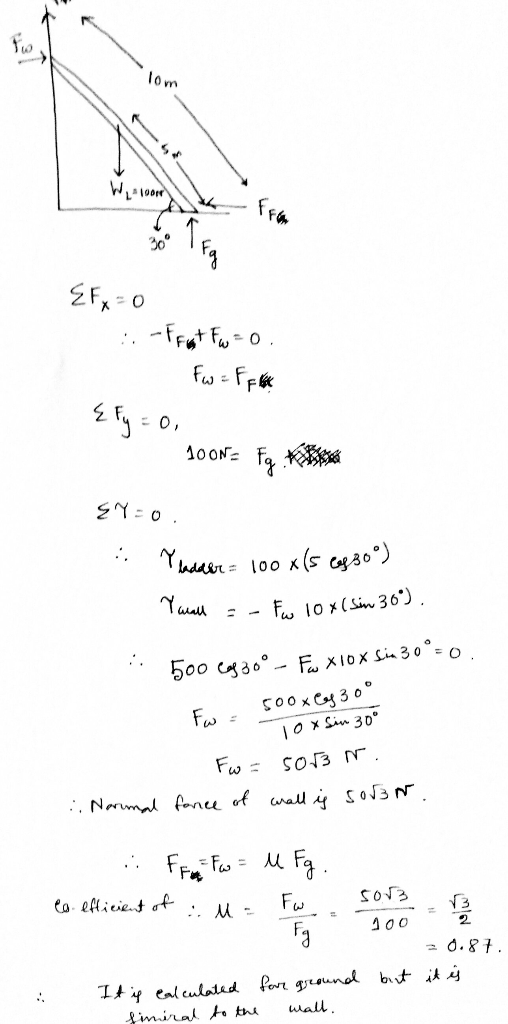#### Earn Coins

Coins can be redeemed for fabulous gifts.

Similar Homework Help Questions
• ### A 10m long ladder of mass 20kg leans against a smooth vertical wall at an angle of 30 to the wall

A 10m long ladder of mass 20kg leans against a smooth vertical wall at an angle of 30 to the wall. The ground is rough, (a) Calculate the component of the force exerted by the ground on the ladder and wall on the ladder (b) What must be the coefficient of static friction at the base of the ladder if it is not to slip when a 50kg person is 4/5 of the way up the ladder? (c) If coefficient...

• ### A ladder rests against a vertical wall. There is no friction between the wall and the...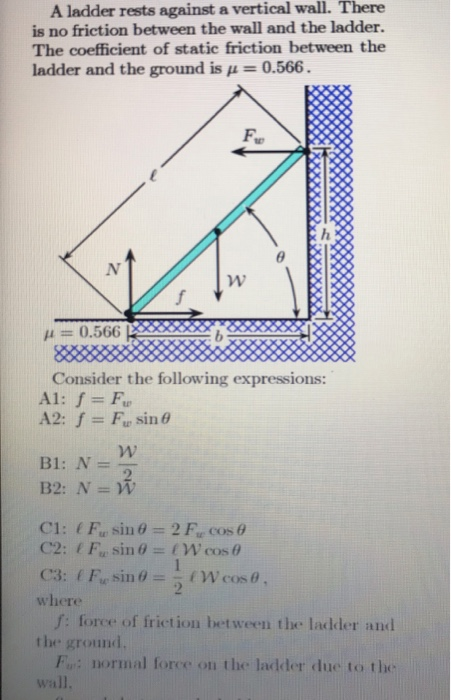A ladder rests against a vertical wall. There is no friction between the wall and the ladder. The coefficient of static friction between the ladder and the ground is μ = 0.566. Fu μ = 0.566 Consider the following expressions: where f: force of friction between the ladder and the ground F: normal force on the ladder due to the where the ground wall, f: force of friction between the ladder and Fu: normal force on the ladder due to...

• ### A 14.0 m uniform ladder weighing 490 N rests against a frictionless wall. The ladder makes...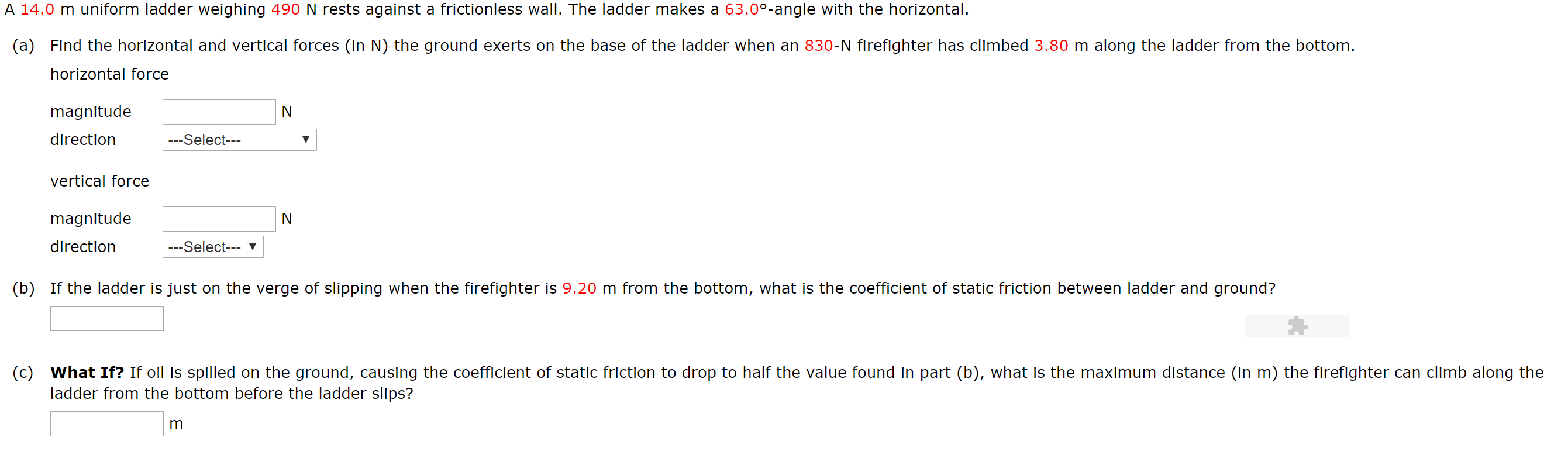A 14.0 m uniform ladder weighing 490 N rests against a frictionless wall. The ladder makes a 63.00-angle with the horizontal. (a) Find the horizontal and vertical forces (in N) the ground exerts on the base of the ladder when an 830-N firefighter has climbed 3.80 m along the ladder from the bottom. horizontal force magnitude direction ---Select--- vertical force magnitude direction ---Select--- V (b) If the ladder is just on the verge of slipping when the firefighter is 9.20...

• ### A 16.0-m uniform ladder weighing 400 N rests against a frictionless wall. The ladder makes an...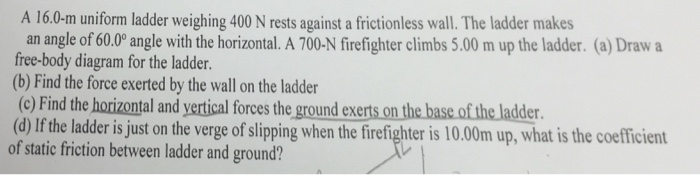A 16.0-m uniform ladder weighing 400 N rests against a frictionless wall. The ladder makes an angle of 60.0 degree angle with the horizontal. A 700-N firefighter climbs 5.00 m up the ladder, Draw a free-body diagram for the ladder. Find the force exerted by the wall on the ladder If the ladder is just on the verge of slipping when the firefighter is 10.00m up, what is the coefficient of static friction between ladder and ground?

• ### A 100N uniform ladder, 8.0m long, rests against a smooth vertical wall. The coefficient of static...

A 100N uniform ladder, 8.0m long, rests against a smooth vertical wall. The coefficient of static friction between the ladder and the floor is 0.40. What minimum angle can the ladder make with the floor before it slips? OPTIONS 22˚ 51˚ 18˚ 42˚

• ### A 16.0 m uniform ladder weighing 480 N rests against a frictionless wall. The ladder makes...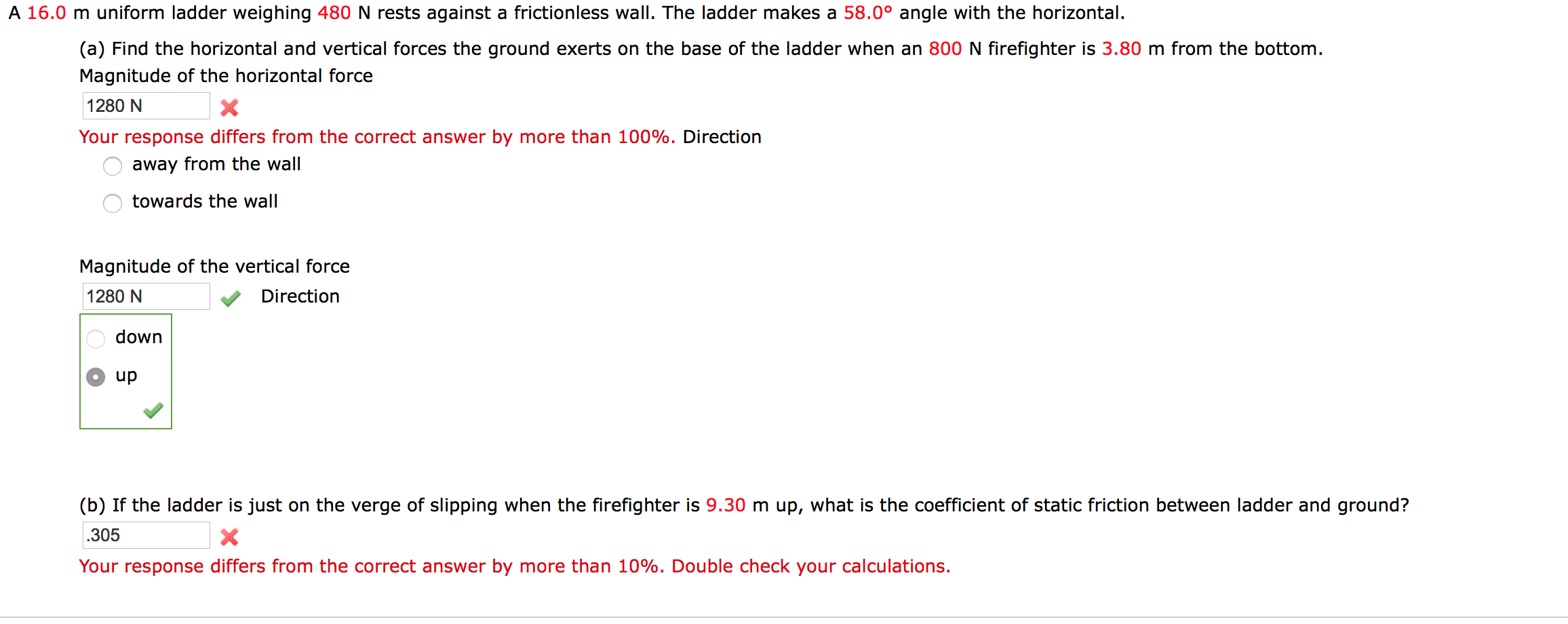A 16.0 m uniform ladder weighing 480 N rests against a frictionless wall. The ladder makes a 58.0 degrees angle with the horizontal. Find the horizontal and vertical forces the ground exerts on the base of the ladder when an 800 N firefighter is 3.80 m from the bottom. Magnitude of the horizontal force Your response differs from the correct answer by more than 100%. Direction away from the wall towards the wall Magnitude of the vertical force If the...

• ### A 14.0-m uniform ladder weighing 520 N rests against a frictionless wall. The ladder makes a...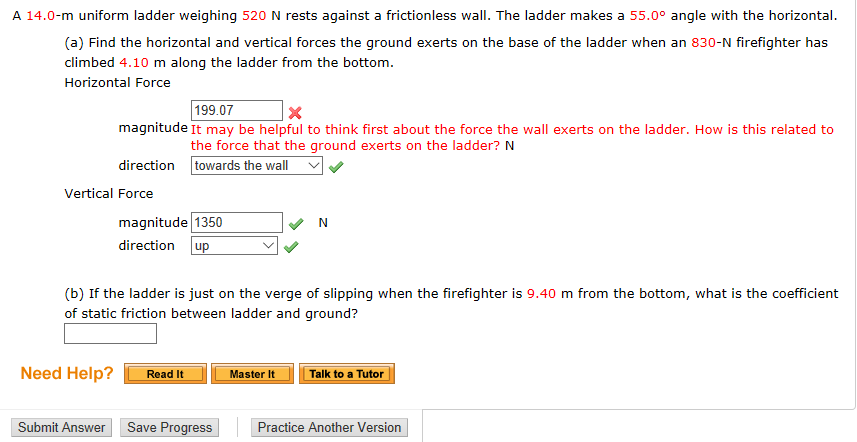A 14.0-m uniform ladder weighing 520 N rests against a frictionless wall. The ladder makes a 55.0degree angle with the horizontal. Find the horizontal and vertical forces the ground exerts on the base of the ladder when an 830-N firefighter has climbed 4.10 m along the ladder from the bottom. Horizontal Force If the ladder is just on the verge of slipping when the firefighter is 9.40 m from the bottom, what is the coefficient of static friction between ladder...

• ### A uniform ladder weighing 186 N is leaning against a wall. The ladder slips when its...

A uniform ladder weighing 186 N is leaning against a wall. The ladder slips when its angle between ladder and the floor is 37◦. The acceleration of gravity is 9.8 m/s2 . Assuming the coefficients of static friction at the wall and the ground are the same, obtain a value for μs. Length of ladder: 15 m

• ### A 75.0 kg ladder that is 3.00 m in length is placed against a wall at an angle theta

A 75.0 kg ladder that is 3.00 m in length is placed against a wall at an angle theta. The center of gravity of the ladder is at a point 1.20 m from the base of the ladder. The coefficient of static friction at the base of the ladder is 0.800. There is no friction between the wall and the ladder. What is the vertical force of the ground on the ladder?

• ### 11.4) A 30-ft ladder weighing 40-lbf is initially stationary and leaning against a wall with θ...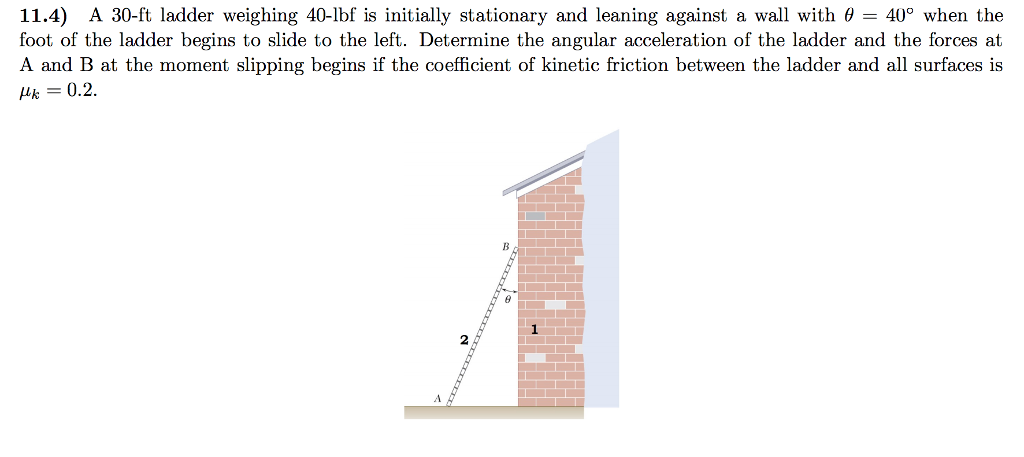11.4) A 30-ft ladder weighing 40-lbf is initially stationary and leaning against a wall with θ 400 when the foot of the ladder begins to slide to the left. Determine the angular acceleration of the ladder and the forces at A and B at the moment slipping begins if the coefficient of kinetic friction between the ladder and all surfaces is k-0.2 2